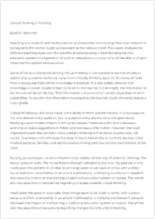# Executive Summary of Pepsico (Finance) 1775

Available Only on StudyHippo
• Pages: 2 (553 words)
• Published: November 1, 2018
Text preview

Through my research of Pepsico, I have calculated the cost of capital. A firm”s cost of capital is imperative because it represents the funds used to finance the firm”s assets and operations. First you have to estimate the cost of capital in order to minimize it.

In estimating the cost of capital, you first have to find the cost of each capital component and then combine the component costs to find the weighted average cost of capital. First, I calculated the cost of debt. Pepsico”s bond consisted of 7 5/8 coupon rate, maturing in 1998 at a price of \$1023.80. I figured the payments to be \$38.15(.0763*1000/2). I then used my financial calculator to find the bond yield of 5.16% by entering in 1023.80=PV, 1000=FV, 2=N, 38.15=PMT. The bond was calculated semi-annually, therefore I multiplied the answer for I/Y times 2 to get 5.16%.

The next step would be to calculate the preferred stock, however my stock had none. I then went to the third step of calculating cost of retained earnings. First I found the three growth rates which were historical, forecast, and sustainable growth. The historical and forecast annual rates I simply pulled directly from Value Line under Past 10 years and estimated years of the dividends. They both were 14.0%. The sustainable growth is calculated by taking the retention rate (b) and multiplying it by the return on equity (r ). To find b, I first calculated the dividends payout ratio which is DPS/EPS. I pulled DPS and EPS fro

...

m value line under 1997. Then to find the retention rate, I subtracted the ratio from 1. Next, I calculated r, by taking net income and dividing it by net worth. These figures I also pulled from Value Line. My b=.352, and r=28.68%. Then the third growth rate was 10.10(.352*28.68).

Still calculating the cost of retained earnings, I then calculated my cash flows by the discounted cash flow approach. For the first three cash flows, I took the dividend of the stock over the price of the stock, and then added the growth rate to it. My first cash flow equaled to 15.38%, second was also 15.38%, and the third one was 11.45%. To find the cash flow four, I used the CAPM approach. This formula is Ks=Krf + (Km-Krf)bs. I found beta on Value Line which was .95. The risk free rate was found by obtaining the current yield on a 20yr. T-bond from the Wall Street Journal. It equaled 6.60%. The Km-Krf was found in the book, and equaled 7.1%. After plugging those numbers into the formula, I came up with 13.35%.

The last calculating was the weighted average cost of capital. The formula for this is: WACC=WdKd(1-tax rate) + WpKpf + WsKs. I found the weights of debt and equity on Value Line, and they were: Wd=55%, and Ws=45%. The cost of debt and equity were already calculated, and the tax rate of 40% was given to us. There was no preferred stock, so I did not use those numbers in my calculation. After plugging in the values, my WACC came out to be 7.71%.

This number can be interprete

Join StudyHippo to see entire essay
Join StudyHippo to see entire essay

as the weighted average cost of each new dollar of capital raised at the margin. The capital is basically the entire right hand side of the balance sheet, and the cost of capital must be minimized in order to maximize the value of the firm.

Join StudyHippo to see entire essay